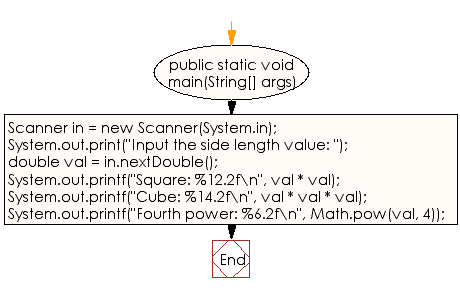﻿ Java exercises: Reads a number and display the square, cube, and fourth power - w3resource# Java Data Type Exercises: Reads a number and display the square, cube, and fourth power

## Java Data Type: Exercise-8 with Solution

Write a Java program that reads a number and display the square, cube, and fourth power.

Test Data
Input the side length value: 15

Sample Solution:

Java Code:

``````import java.util.Scanner;
public class Exercise8 {

public static void main(String[] args)
{
Scanner in = new Scanner(System.in);
System.out.print("Input the side length value: ");
double val = in.nextDouble();

System.out.printf("Square: %12.2f\n", val * val);
System.out.printf("Cube: %14.2f\n", val * val * val);
System.out.printf("Fourth power: %6.2f\n", Math.pow(val, 4));
}
}
```
```

Sample Output:

```Input the side length value: 15
Square: .2f
Cube: .2f
Fourth power: 50625.00
```

Flowchart:JavaCode Editor:

Improve this sample solution and post your code through Disqus

What is the difficulty level of this exercise?

﻿

## Java: Tips of the Day

Checks if a string is upper case:

```public static boolean isUpperCase(String input) {
return Objects.equals(input, input.toUpperCase());
}
```

Ref: https://bit.ly/39Hpo84